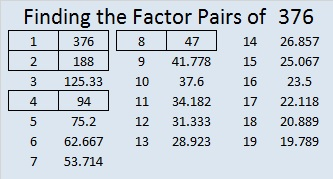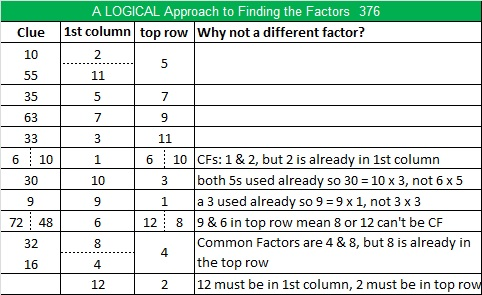# 376 Happy Birthday, Doug

I hope you have a wonderful day, Doug.

In our family we were the closest in age, so we often went to the same school. He was brilliant in mathematics, and I wanted to follow in his footsteps. Before I started this blog, I showed him and his wife a few Find the Factors puzzles. They were both very encouraging. We live thousands of miles away from each other, but I am grateful we were able to spend time with each other twice in 2014.

Happy birthday, Doug!Print the puzzles or type the factors on this excel file: 10 Factors 2015-02-02

• 376 is a composite number.
• Prime factorization: 376 = 2 x 2 x 2 x 47, which can be written 376 = (2^3) x 47
• The exponents in the prime factorization are 3 and 1. Adding one to each and multiplying we get (3 + 1)(1 + 1) = 4 x 2 = 8. Therefore 376 has exactly 8 factors.
• Factors of 376: 1, 2, 4, 8, 47, 94, 188, 376
• Factor pairs: 376 = 1 x 376, 2 x 188, 4 x 94, or 8 x 47
• Taking the factor pair with the largest square number factor, we get √376 = (√4)(√94) = 2√94 ≈ 19.391Here’s an interesting tweet about the number 376: